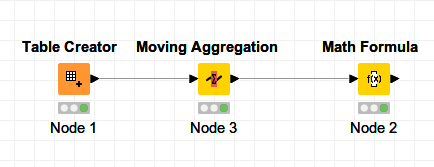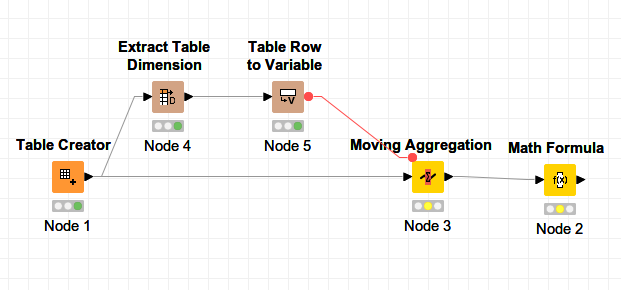# How to calculate data for burndown chart?

I have a bunch of ordered (by timestamp) values

``````1.1  | 10
1.2. | 20
1.3. | 15
1.4. | 10
1.5. | 15
1.6. | 20
1.7. | 10
``````

I would like to display the data in a kind of burndown chart. So first I need the sum of the values (in this case 100). That’s quite easy.
But now I need the difference from the sum minus the sum of values “before”

``````1.1. | 10   | 100  | 90
1.2. | 20   | 100  | 70
1.3. | 15   | 100  | 55
1.4. | 10   | 100  | 45
1.5. | 15   | 100  | 30
1.6. | 20   | 100  | 10
1.7. | 10   | 100  | 0
``````

At the moment I have absolutly no clue what nodes can help me to make the calculation of cloumn 4.
Is there a nice node which can help me to do this job?

For the solution I used 2 nodes, see calculate_for_burndown_chart.knwf (14.7 KB).At least it worksBut I’m sure somebody else can do it in just 1 node.
gr. Hans

2 Likes

Hello HansS,

that works.
But there is only one pitfall which causes some issues to me: This nodes calculates the average/sum/… over a defined amount of rows. the amount is part of the configuration. The amount can only be the count of rows. If it is higher the node throws an error.
If there is no chance to have a good estimate of the amount of data points, it does not work fine.

Is there a node which can count the rows in table? Assigning that result to a variable and use it in the moving aggregartion node could overcome that issue.

1 Like

Hi @knimediger,

I don’t understand your question so well I think. But with Extract Table Dimension, you are able to extract the number of rows in your table. Then you can convert to a flow variable, and use the number of rows in the Moving Average node (or any other node). Hope this helps.gr.Hans

3 Likes

Hello @HansS,

the https://nodepit.com/node/org.knime.base.node.util.extracttabledimension.ExtractTableDimensionNodeFactory solves my last question. Using the flow variable with the row count I’m able to calculate the sum exactly over the whole table and reduce the amount by each entry.

4 Likes

This topic was automatically closed 7 days after the last reply. New replies are no longer allowed.